Study Materials: ncert solutions

Our ncert solutions for Exercise 2.3 Class 11 maths 2. Relations and Functions - ncert solutions - Toppers Study is the best material for English Medium students cbse board and other state boards students.

Solutions ⇒ Class 11th ⇒ Mathematics ⇒ 2. Relations and Functions

# Exercise 2.3 Class 11 maths 2. Relations and Functions - ncert solutions - Toppers Study

Topper Study classes prepares ncert solutions on practical base problems and comes out with the best result that helps the students and teachers as well as tutors and so many ecademic coaching classes that they need in practical life. Our ncert solutions for Exercise 2.3 Class 11 maths 2. Relations and Functions - ncert solutions - Toppers Study is the best material for English Medium students cbse board and other state boards students.

## Exercise 2.3 Class 11 maths 2. Relations and Functions - ncert solutions - Toppers Study

CBSE board students who preparing for class 11 ncert solutions maths and Mathematics solved exercise chapter 2. Relations and Functions available and this helps in upcoming exams 2023-2024.

### You can Find Mathematics solution Class 11 Chapter 2. Relations and Functions

• All Chapter review quick revision notes for chapter 2. Relations and Functions Class 11
• NCERT Solutions And Textual questions Answers Class 11 Mathematics
• Extra NCERT Book questions Answers Class 11 Mathematics
• Importatnt key points with additional Assignment and questions bank solved.

NCERT Solutions do not only help you to cover your syllabus but also will give to textual support in exams 2023-2024 to complete exercise 2.3 maths class 11 chapter 2 in english medium. So revise and practice these all cbse study materials like class 11 maths chapter 2.3 in english ncert book. Also ensure to repractice all syllabus within time or before board exams for ncert class 11 maths ex 2.3 in english.

See all solutions for class 11 maths chapter 2 exercise 2 in english medium solved questions with answers.

### Exercise 2.3 class 11 Mathematics Chapter 2. Relations and Functions

• Exercise 2.3 Class 11 Maths 2. Relations And Functions - Ncert Solutions - Toppers Study
• Class 11 Ncert Solutions
• Solution Chapter 2. Relations And Functions Class 11
• Solutions Class 11
• Chapter 2. Relations And Functions Exercise 2.3 Class 11

## Exercise 2.3 Class 11 maths 2. Relations and Functions - ncert solutions - Toppers Study

Exercise 2.3

Q1. Which of the following relations are functions? Give reasons. If it is a function, determine its domain and range.
(i) {(2,1), (5,1), (8,1), (11,1), (14,1), (17,1)}
(ii) {(2,1), (4,2), (6,3), (8,4), (10,5), (12,6), (14,7)}
(iii) {(1,3), (1,5), (2,5)}.

Solution:

(i) {(2, 1), (5, 1), (8, 1), (11, 1), (14, 1), (17, 1)}

Let be R = {(2, 1), (5, 1), (8, 1), (11, 1), (14, 1), (17, 1)}

There is no same component in the domain of given relation, so this relation is a function.

Here, domain = {2, 5, 8, 11, 14, 17} and range = {1}

Solution:

(ii) {(2, 1), (4, 2), (6, 3), (8, 4), (10, 5), (12, 6), (14, 7)}

Let be R = {(2, 1), (4, 2), (6, 3), (8, 4), (10, 5), (12, 6), (14, 7)}

There are also unique domain components or order pair in the given relation, so this relation is a function.

Here, domain = {2, 4, 6, 8, 10, 12, 14} and range = {1, 2, 3, 4, 5, 6, 7}

Solution:

(iii) {(1, 3), (1, 5), (2, 5)}

Since the domain has the same first element i.e., 1 corresponds to two different images i.e.,

3 and 5, this relation is not a function.

Q2. Find the domain and range of the following real functions:

(i) f(x) = – |x|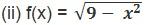Solution2: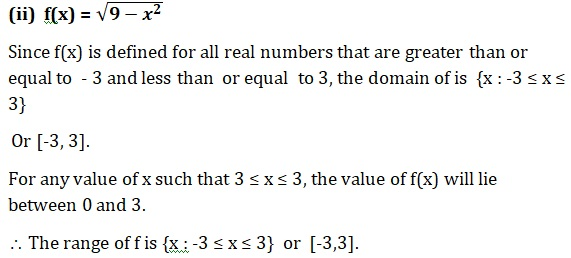Q3:  A function f is defined by f(x) = 2x – 5

(i) f (0)                      (ii) f(7)                        (iii) f(-3)

Solution 3:

The given function is f(x) = 2x – 5

Therefore,

(i) f(0) = 2 × 0 – 5 = 0 – 5 = - 5

(ii) f(7) = 2 × 7 – 5 = 14 – 5 = - 9

(iii) f(-3) = 2 × -3 – 5 = -6 – 5 = - 11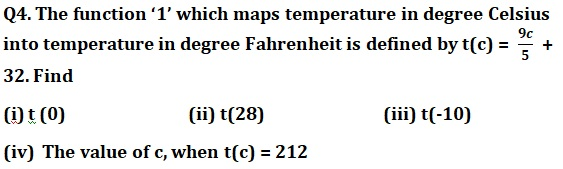Solution: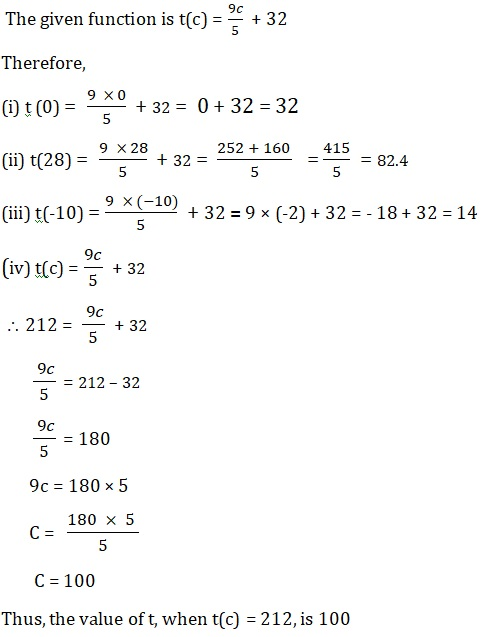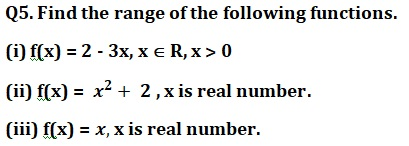Solution: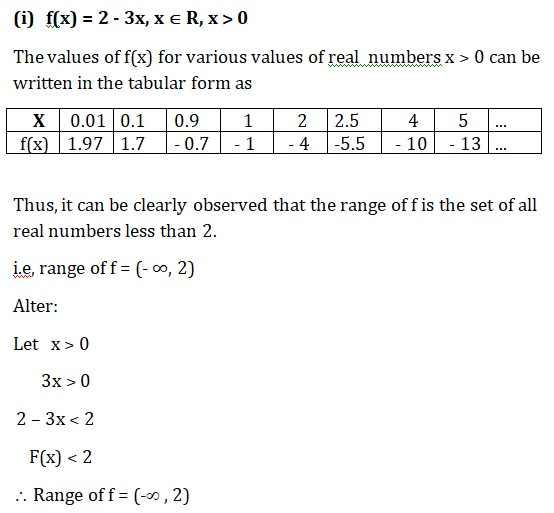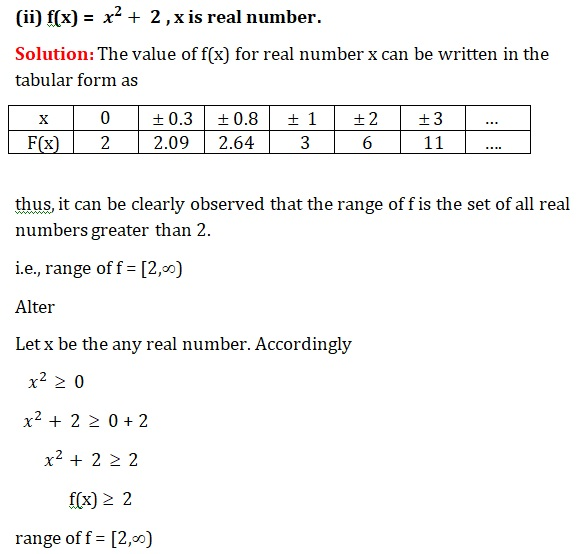(iii) f(x) = x is real number.

It is clear that the range of f is the set of all real number.

Therefore,  range of f = R

##### Other Pages of this Chapter: 2. Relations and Functions

Important Study materials for classes 06, 07, 08,09,10, 11 and 12. Like CBSE Notes, Notes for Science, Notes for maths, Notes for Social Science, Notes for Accountancy, Notes for Economics, Notes for political Science, Noes for History, Notes For Bussiness Study, Physical Educations, Sample Papers, Test Papers, Mock Test Papers, Support Materials and Books.Mathematics Class - 11th

NCERT Maths book for CBSE Students.

books

## Study Materials List:

##### Solutions ⇒ Class 11th ⇒ Mathematics
1. Sets
2. Relations and Functions
3. Trigonometric Functions
4. Principle Of Mathematical Induction
5. Complex Numbers and Quadratic Equations
6. Linear Inequalities
7. Permutations and Combinations
8. Binomial Theorem
9. Sequences and Series
10. Straight Lines
11. Conic Sections
12. Introduction to Three Dimensional Geometry
13. Limits and Derivatives
14. Mathematical Reasoning
15. Statistics
16. Probability

New Books